# ksdensity

Kernel smoothing function estimate for univariate and bivariate data

## Syntax

``````[f,xi] = ksdensity(x)``````
``````[f,xi] = ksdensity(x,pts)``````
``````[f,xi] = ksdensity(___,Name,Value)``````
``````[f,xi,bw] = ksdensity(___)``````
``ksdensity(___)``
``ksdensity(ax,___)``

## Description

example

``````[f,xi] = ksdensity(x)``` returns a probability density estimate, `f`, for the sample data in the vector or two-column matrix `x`. The estimate is based on a normal kernel function, and is evaluated at equally-spaced points, `xi`, that cover the range of the data in `x`. `ksdensity` estimates the density at 100 points for univariate data, or 900 points for bivariate data.`ksdensity` works best with continuously distributed samples.```

example

``````[f,xi] = ksdensity(x,pts)``` specifies points (`pts`) to evaluate `f`. Here, `xi` and `pts` contain identical values.```

example

``````[f,xi] = ksdensity(___,Name,Value)``` uses additional options specified by one or more name-value pair arguments in addition to any of the input arguments in the previous syntaxes. For example, you can define the function type `ksdensity` evaluates, such as probability density, cumulative probability, survivor function, and so on. Or you can specify the bandwidth of the smoothing window.```

example

``````[f,xi,bw] = ksdensity(___)``` also returns the bandwidth of the kernel smoothing window, `bw`. The default bandwidth is the optimal for normal densities.```

example

````ksdensity(___)` plots the kernel smoothing function estimate.```
````ksdensity(ax,___)` plots the results using axes with the handle, `ax`, instead of the current axes returned by `gca`.```

## Examples

collapse all

Generate a sample data set from a mixture of two normal distributions.

```rng('default') % For reproducibility x = [randn(30,1); 5+randn(30,1)];```

Plot the estimated density.

```[f,xi] = ksdensity(x); figure plot(xi,f);```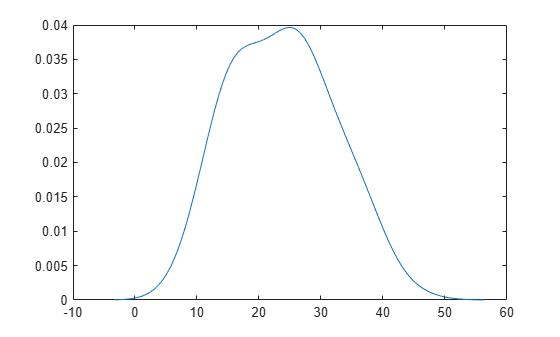The density estimate shows the bimodality of the sample.

Generate a nonnegative sample data set from the half-normal distribution.

```rng('default') % For reproducibility pd = makedist('HalfNormal','mu',0,'sigma',1); x = random(pd,100,1);```

Estimate pdfs with two different boundary correction methods, log transformation and reflection, by using the `'BoundaryCorrection'` name-value pair argument.

```pts = linspace(0,5,1000); % points to evaluate the estimator [f1,xi1] = ksdensity(x,pts,'Support','positive'); [f2,xi2] = ksdensity(x,pts,'Support','positive','BoundaryCorrection','reflection');```

Plot the two estimated pdfs.

```plot(xi1,f1,xi2,f2) lgd = legend('log','reflection'); title(lgd, 'Boundary Correction Method') xl = xlim; xlim([xl(1)-0.25 xl(2)])```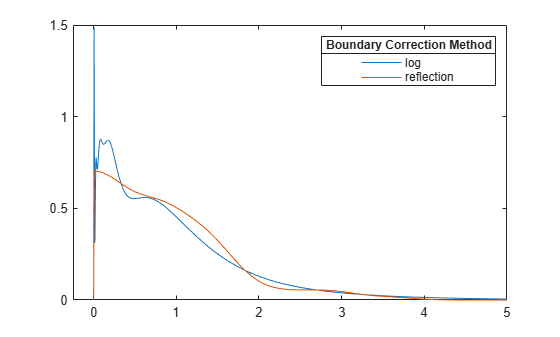`ksdensity` uses a boundary correction method when you specify either positive or bounded support. The default boundary correction method is log transformation. When `ksdensity` transforms the support back, it introduces the `1/x` term in the kernel density estimator. Therefore, the estimate has a peak near `x = 0`. On the other hand, the reflection method does not cause undesirable peaks near the boundary.

`load hospital`

Compute and plot the estimated cdf evaluated at a specified set of values.

```pts = (min(hospital.Weight):2:max(hospital.Weight)); figure() ecdf(hospital.Weight) hold on [f,xi,bw] = ksdensity(hospital.Weight,pts,'Support','positive',... 'Function','cdf'); plot(xi,f,'-g','LineWidth',2) legend('empirical cdf','kernel-bw:default','Location','northwest') xlabel('Patient weights') ylabel('Estimated cdf')```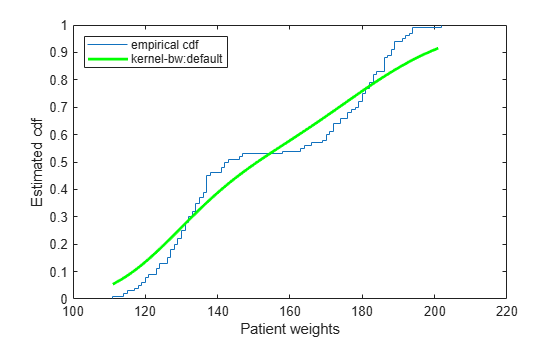`ksdensity` seems to smooth the cumulative distribution function estimate too much. An estimate with a smaller bandwidth might produce a closer estimate to the empirical cumulative distribution function.

Return the bandwidth of the smoothing window.

`bw`
```bw = 0.1070 ```

Plot the cumulative distribution function estimate using a smaller bandwidth.

```[f,xi] = ksdensity(hospital.Weight,pts,'Support','positive',... 'Function','cdf','Bandwidth',0.05); plot(xi,f,'--r','LineWidth',2) legend('empirical cdf','kernel-bw:default','kernel-bw:0.05',... 'Location','northwest') hold off```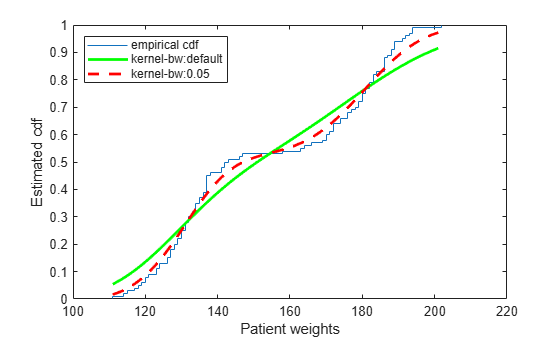The `ksdensity` estimate with a smaller bandwidth matches the empirical cumulative distribution function better.

`load hospital`

Plot the estimated cdf evaluated at 50 equally spaced points.

```figure() ksdensity(hospital.Weight,'Support','positive','Function','cdf',... 'NumPoints',50) xlabel('Patient weights') ylabel('Estimated cdf')```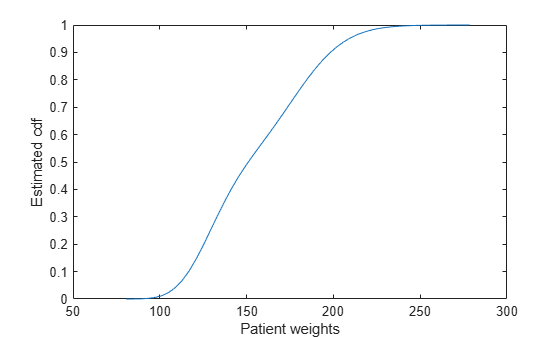Generate sample data from an exponential distribution with mean 3.

```rng('default') % For reproducibility x = random('exp',3,100,1);```

Create a logical vector that indicates censoring. Here, observations with lifetimes longer than 10 are censored.

```T = 10; cens = (x>T);```

Compute and plot the estimated density function.

```figure ksdensity(x,'Support','positive','Censoring',cens);```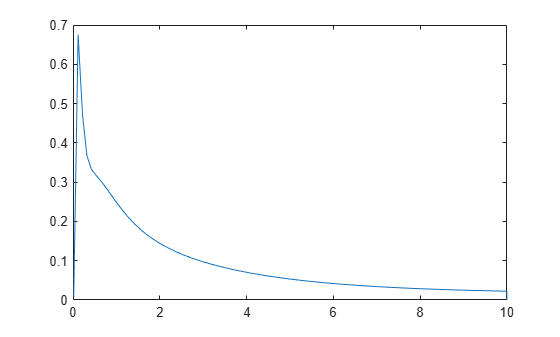Compute and plot the survivor function.

```figure ksdensity(x,'Support','positive','Censoring',cens,... 'Function','survivor');```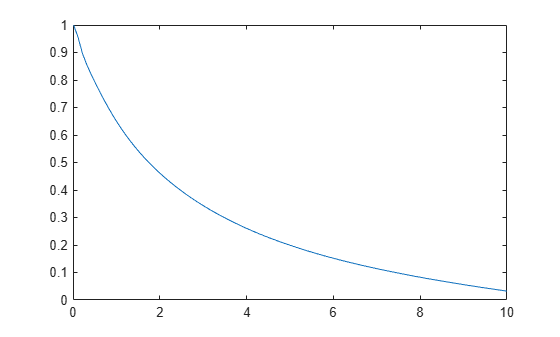Compute and plot the cumulative hazard function.

```figure ksdensity(x,'Support','positive','Censoring',cens,... 'Function','cumhazard');```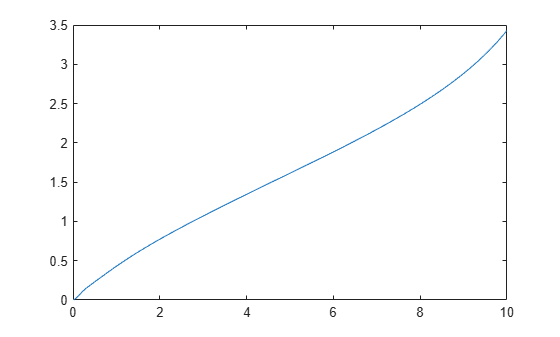Generate a mixture of two normal distributions, and plot the estimated inverse cumulative distribution function at a specified set of probability values.

```rng('default') % For reproducibility x = [randn(30,1); 5+randn(30,1)]; pi = linspace(.01,.99,99); figure ksdensity(x,pi,'Function','icdf');```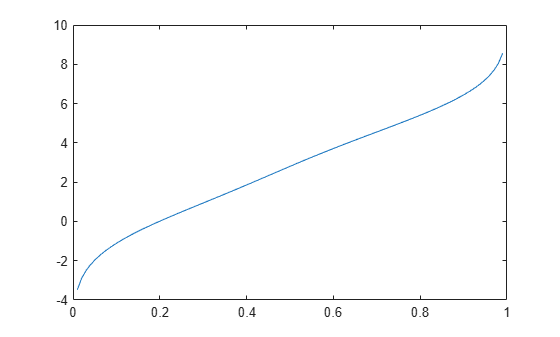Generate a mixture of two normal distributions.

```rng('default') % For reproducibility x = [randn(30,1); 5+randn(30,1)];```

Return the bandwidth of the smoothing window for the probability density estimate.

```[f,xi,bw] = ksdensity(x); bw```
```bw = 1.5141 ```

The default bandwidth is optimal for normal densities.

Plot the estimated density.

```figure plot(xi,f); xlabel('xi') ylabel('f') hold on```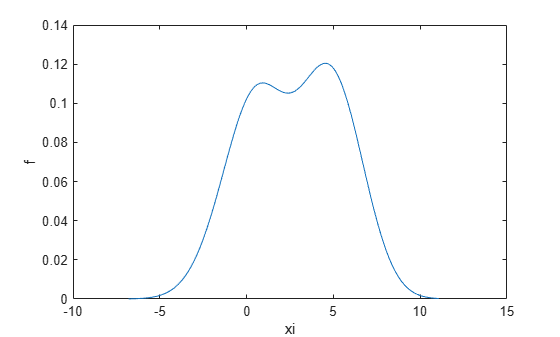Plot the density using an increased bandwidth value.

```[f,xi] = ksdensity(x,'Bandwidth',1.8); plot(xi,f,'--r','LineWidth',1.5)```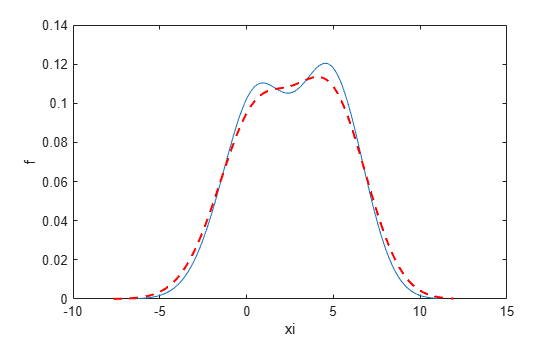A higher bandwidth further smooths the density estimate, which might mask some characteristics of the distribution.

Now, plot the density using a decreased bandwidth value.

```[f,xi] = ksdensity(x,'Bandwidth',0.8); plot(xi,f,'-.k','LineWidth',1.5) legend('bw = default','bw = 1.8','bw = 0.8') hold off```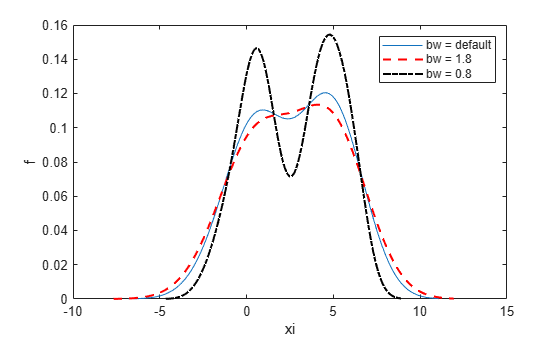A smaller bandwidth smooths the density estimate less, which exaggerates some characteristics of the sample.

Create a two-column vector of points at which to evaluate the density.

```gridx1 = -0.25:.05:1.25; gridx2 = 0:.1:15; [x1,x2] = meshgrid(gridx1, gridx2); x1 = x1(:); x2 = x2(:); xi = [x1 x2];```

Generate a 30-by-2 matrix containing random numbers from a mixture of bivariate normal distributions.

```rng('default') % For reproducibility x = [0+.5*rand(20,1) 5+2.5*rand(20,1); .75+.25*rand(10,1) 8.75+1.25*rand(10,1)];```

Plot the estimated density of the sample data.

```figure ksdensity(x,xi);```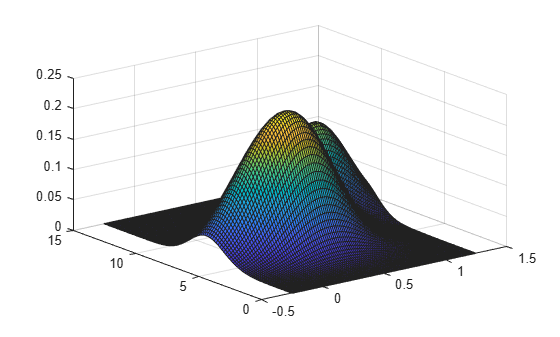## Input Arguments

collapse all

Sample data for which `ksdensity` returns `f` values, specified as a column vector or two-column matrix. Use a column vector for univariate data, and a two-column matrix for bivariate data.

Example: `[f,xi] = ksdensity(x)`

Data Types: `single` | `double`

Points at which to evaluate `f`, specified as a vector or two-column matrix. For univariate data, `pts` can be a row or column vector. The length of the returned output `f` is equal to the number of points in `pts`.

Example: ```pts = (0:1:25); ksdensity(x,pts);```

Data Types: `single` | `double`

Axes handle for the figure `ksdensity` plots to, specified as a handle.

For example, if `h` is a handle for a figure, then `ksdensity` can plot to that figure as follows.

Example: `ksdensity(h,x)`

### Name-Value Pair Arguments

Specify optional comma-separated pairs of `Name,Value` arguments. `Name` is the argument name and `Value` is the corresponding value. `Name` must appear inside quotes. You can specify several name and value pair arguments in any order as `Name1,Value1,...,NameN,ValueN`.

Example: `'Censoring',cens,'Kernel','triangle','NumPoints',20,'Function','cdf'` specifies that `ksdensity` estimates the cdf by evaluating at 20 equally spaced points that covers the range of data, using the triangle kernel smoothing function and accounting for the censored data information in vector `cens`.

The bandwidth of the kernel-smoothing window, which is a function of the number of points in `x`, specified as the comma-separated pair consisting of `'Bandwidth'` and a scalar value. If the sample data is bivariate, `Bandwidth` can also be a two-element vector. The default is optimal for estimating normal densities , but you might want to choose a larger or smaller value to smooth more or less.

If you specify `'BoundaryCorrection'` as `'log'`(default) and `'Support'` as either `'positive'` or a vector ```[L U]```, `ksdensity` converts bounded data to be unbounded by using log transformation. The value of `'Bandwidth'` is on the scale of the transformed values.

Example: `'Bandwidth',0.8`

Data Types: `single` | `double`

Boundary correction method, specified as the comma-separated pair consisting of `'BoundaryCorrection'` and `'log'` or `'reflection'`.

ValueDescription
`'log'`

`ksdensity` converts bounded data `x` to be unbounded by one of the following transformations. Then, it transforms back to the original bounded scale after density estimation.

• For univariate data, if you specify `'Support','positive'`, then `ksdensity` applies `log(x)`.

• For univariate data, if you specify `'Support',[L U]`, where `L` and `U` are numeric scalars and `L < U`, then `ksdensity` applies `log((x-L)/(U–x))`.

• For bivariate data, `ksdensity` transforms each column of `x` in the same way with the univariate data.

The value of `'Bandwidth'` and the `bw` output are on the scale of the transformed values.

`'reflection'`

`ksdensity` augments bounded data by adding reflected data near the boundaries, then it returns estimates corresponding to the original support. For details, see Reflection Method.

`ksdensity` applies boundary correction only when you specify `'Support'` as a value other than `'unbounded'`.

Example: `'BoundaryCorrection','reflection'`

Logical vector indicating which entries are censored, specified as the comma-separated pair consisting of `'Censoring'` and a vector of binary values. A value of 0 indicates there is no censoring, 1 indicates that observation is censored. Default is there is no censoring. This name-value pair is only valid for univariate data.

Example: `'Censoring',censdata`

Data Types: `logical`

Function to estimate, specified as the comma-separated pair consisting of `'Function'` and one of the following.

ValueDescription
`'pdf'`Probability density function.
`'cdf'`Cumulative distribution function.
`'icdf'`

Inverse cumulative distribution function. `ksdensity` computes the estimated inverse cdf of the values in `x`, and evaluates it at the probability values specified in `pi`.

This value is valid only for univariate data.

`'survivor'`Survivor function.
`'cumhazard'`

Cumulative hazard function.

This value is valid only for univariate data.

Example: `'Function'`,`'icdf'`

Type of kernel smoother, specified as the comma-separated pair consisting of `'Kernel'` and one of the following.

• `'normal'` (default)

• `'box'`

• `'triangle'`

• `'epanechnikov'`

• A kernel function that is a custom or built-in function. Specify the function as a function handle (for example, `@myfunction` or `@normpdf`) or as a character vector or string scalar (for example, `'myfunction'` or `'normpdf'`). The software calls the specified function with one argument that is an array of distances between data values and locations where the density is evaluated. The function must return an array of the same size containing corresponding values of the kernel function.

When `'Function'` is `'pdf'`, the kernel function returns density values. Otherwise, it returns cumulative probability values.

Specifying a custom kernel when `'Function'` is `'icdf'` returns an error.

For bivariate data, `ksdensity` applies the same kernel to each dimension.

Example: `'Kernel','box'`

Number of equally spaced points in `xi`, specified as the comma-separated pair consisting of `'NumPoints'` and a scalar value. This name-value pair is only valid for univariate data.

For example, for a kernel smooth estimate of a specified function at 80 equally spaced points within the range of sample data, input:

Example: `'NumPoints',80`

Data Types: `single` | `double`

Support for the density, specified as the comma-separated pair consisting of `'support'` and one of the following.

ValueDescription
`'unbounded'`Default. Allow the density to extend over the whole real line.
`'positive'`Restrict the density to positive values.
Two-element vector, `[L U]`Give the finite lower and upper bounds for the support of the density. This option is only valid for univariate sample data.
Two-by-two matrix, ```[L1 L2; U1 U2]```Give the finite lower and upper bounds for the support of the density. The first row contains the lower limits and the second row contains the upper limits. This option is only valid for bivariate sample data.

For bivariate data, `'Support'` can be a combination of positive, unbounded, or bounded variables specified as ```[0 -Inf; Inf Inf]``` or `[0 L; Inf U]`.

Example: `'Support','positive'`

Example: `'Support',[0 10]`

Data Types: `single` | `double` | `char` | `string`

Function used to create kernel density plot, specified as the comma-separated pair consisting of `'PlotFcn'` and one of the following.

ValueDescription
`'surf'`3-D shaded surface plot, created using `surf`
`'contour'`Contour plot, created using `contour`
`'plot3'`3-D line plot, created using `plot3`
`'surfc'`Contour plot under a 3-D shaded surface plot, created using `surfc`

This name-value pair is only valid for bivariate sample data.

Example: `'PlotFcn','contour'`

Weights for sample data, specified as the comma-separated pair consisting of `'Weights'` and a vector of length `size(x,1)`, where `x` is the sample data.

Example: `'Weights',xw`

Data Types: `single` | `double`

## Output Arguments

collapse all

Estimated function values, returned as a vector whose length is equal to the number of points in `xi` or `pts`.

Evaluation points at which `ksdensity` calculates `f`, returned as a vector or a two-column matrix. For univariate data, the default is 100 equally-spaced points that cover the range of data in `x`. For bivariate data, the default is 900 equally-spaced points created using `meshgrid` from 30 equally-spaced points in each dimension.

Bandwidth of smoothing window, returned as a scalar value.

If you specify `'BoundaryCorrection'` as `'log'`(default) and `'Support'` as either `'positive'` or a vector `[L U]`, `ksdensity` converts bounded data to be unbounded by using log transformation. The value of `bw` is on the scale of the transformed values.

collapse all

### Kernel Distribution

A kernel distribution is a nonparametric representation of the probability density function (pdf) of a random variable. You can use a kernel distribution when a parametric distribution cannot properly describe the data, or when you want to avoid making assumptions about the distribution of the data. A kernel distribution is defined by a smoothing function and a bandwidth value, which control the smoothness of the resulting density curve.

The kernel density estimator is the estimated pdf of a random variable. For any real values of x, the kernel density estimator's formula is given by

`${\stackrel{^}{f}}_{h}\left(x\right)=\frac{1}{nh}\sum _{i=1}^{n}K\left(\frac{x-{x}_{i}}{h}\right)\text{\hspace{0.17em}},$`

where x1, x2, …, xn are random samples from an unknown distribution, n is the sample size, $K\left(·\right)$ is the kernel smoothing function, and h is the bandwidth.

The kernel estimator for the cumulative distribution function (cdf), for any real values of x, is given by

`${\stackrel{^}{F}}_{h}\left(x\right)={\int }_{-\infty }^{x}{\stackrel{^}{f}}_{h}\left(t\right)dt=\frac{1}{n}\sum _{i=1}^{n}G\left(\frac{x-{x}_{i}}{h}\right)\text{\hspace{0.17em}},$`

where $G\left(x\right)={\int }_{-\infty }^{x}K\left(t\right)dt$.

For more details, see Kernel Distribution.

### Reflection Method

The reflection method is a boundary correction method that accurately finds kernel density estimators when a random variable has bounded support. If you specify `'BoundaryCorrection','reflection'`, `ksdensity` uses the reflection method. This method augments bounded data by adding reflected data near the boundaries, and estimates the pdf. Then, `ksdensity` returns the estimated pdf corresponding to the original support with proper normalization, so that the estimated pdf's integral over the original support is equal to one.

If you additionally specify `'Support',[L U]`, then `ksdensity` finds the kernel estimator as follows.

• If `'Function'` is `'pdf'`, then the kernel density estimator is

${\stackrel{^}{f}}_{h}\left(x\right)=\frac{1}{nh}\sum _{i=1}^{n}\left[K\left(\frac{x-{x}_{i}^{-}}{h}\right)+K\left(\frac{x-{x}_{i}}{h}\right)+K\left(\frac{x-{x}_{i}^{+}}{h}\right)\right]$ for L ≤ x ≤ U,

where ${x}_{i}^{-}=2L-{x}_{i}$, ${x}_{i}^{+}=2U-{x}_{i}$, and xi is the `i`th sample data.

• If `'Function'` is `'cdf'`, then the kernel estimator for cdf is

${\stackrel{^}{F}}_{h}\left(x\right)=\frac{1}{n}\sum _{i=1}^{n}\left[G\left(\frac{x-{x}_{i}^{-}}{h}\right)+G\left(\frac{x-{x}_{i}}{h}\right)+G\left(\frac{x-{x}_{i}^{+}}{h}\right)\right]-\frac{1}{n}\sum _{i=1}^{n}\left[G\left(\frac{L-{x}_{i}^{-}}{h}\right)+G\left(\frac{L-{x}_{i}}{h}\right)+G\left(\frac{L-{x}_{i}^{+}}{h}\right)\right]$ for L ≤ x ≤ U.

• To obtain a kernel estimator for an inverse cdf, a survivor function, or a cumulative hazard function (when `'Function'` is `'icdf'`, `'survivor'`, or `'cumhazrd'`), `ksdensity` uses both ${\stackrel{^}{f}}_{h}\left(x\right)$ and ${\stackrel{^}{F}}_{h}\left(x\right)$.

If you additionally specify `'Support'` as `'positive'` or `[0 inf]`, then `ksdensity` finds the kernel estimator by replacing `[L U]` with `[0 inf]` in the above equations.

 Bowman, A. W., and A. Azzalini. Applied Smoothing Techniques for Data Analysis. New York: Oxford University Press Inc., 1997.

 Hill, P. D. “Kernel estimation of a distribution function.” Communications in Statistics - Theory and Methods. Vol 14, Issue. 3, 1985, pp. 605-620.

 Jones, M. C. “Simple boundary correction for kernel density estimation.” Statistics and Computing. Vol. 3, Issue 3, 1993, pp. 135-146.

 Silverman, B. W. Density Estimation for Statistics and Data Analysis. Chapman & Hall/CRC, 1986.

﻿